Density Estimation and Nonparametric Regression

2018-08-14

This vignette will have three main parts. In the first part we will step through two examples of kernel density estimation. In the second part we will step through two examples of non-parametric regression. Finally, in the third part we will show an example that includes UTM conversion and creation of polygons from maps (using packages outside latticeDensity).

Kernel Density Estimation:

latticeDensity is designed to produce density maps accounting for the effects of boundaries and holes. The minimum data needed to perform density estimation in latticeDensity is two 2-column matrices. One gives the vertices of a polygon (clockwise around the interior) and the other the Easting and Northing locations of the observations. Note that an observation outside the boundary polygon is moved automatically to the nearest location inside the polygon.

Step One:

The function nodeFilling takes a polygon representing, in this case, a lake with a causeway and no islands (“holes”“) and fills the region with a grid of equally-spaced nodes. You should select node_spacing to be small, bound only by the speed of processing the data. With small node_spacing, this analysis closely approximates a diffusion.

library(latticeDensity)
library(sp)
plot.new()
data(polygon1)
##           [,1]      [,2]
## [1,] 0.6421053 0.8132050
## [2,] 0.6845247 0.4814305
## [3,] 0.7057345 0.2858322
## [4,] 0.6696779 0.2066025
## [5,] 0.5190888 0.1892710
## [6,] 0.5445405 0.4145805
nodeFillingOutput <- nodeFilling(poly=polygon1, node_spacing=0.02)
plot(nodeFillingOutput)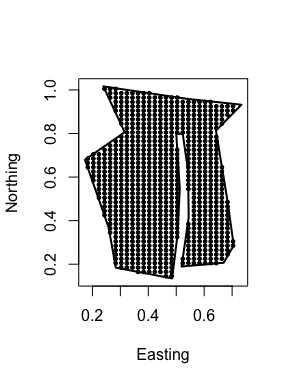Step Two:

Now for the fun part. The function formLattice will ‘connect the dots’ to create a neighbor structure on the nodes. Neighbors are defined to be locations to the N, NE, E, SE, S, SW, W, and NW of each node (as long as there IS a node in that direction). You can examine a plot of the resulting object to see if all regions that should be connected are and that nodes, for instance, across a causeway are not connected.

formLatticeOutput <- formLattice(nodeFillingOutput)
plot(formLatticeOutput)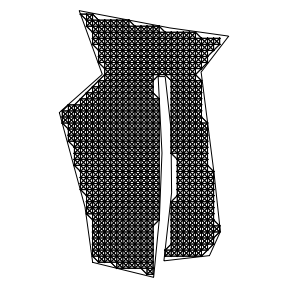Optional Step:

If you see links that you would like to remove (for instance, a link crosses a boundary), or see links that you would like to add, use the function editLattice.

Step Three:

Next, we need to add locations for the point process (e.g. animal relocations) to the lattice object. After the data has been added, we can select a value that represents the number of steps the random walk takes around each observation. These random walks essentially produce a kernel around the observation. There is another argument that we won’t use at this time, . This is the maximum probability that the random walk moves in any single step and is set, by default, to 0.5. For instance, if a node has eight neighbors (the largest possible) and M = 0.5, the flow rate to each neighbor is 0.5/8 = 0.0625. Decreasing this value will decrease the width of a kernel for a fixed step size, so generally it should be decreased when the optimal step size turns out to be so small that large parts of the region are outside any kernel.

Internally, this package uses the function addObservations, but this is called by the functions that we will use, which are createDensity which produces a predicted density surface for a given number of steps , and crossvalDensity which uses crossvalidation over a range of value of to select the optimal step size.

Just for this example I’ll use the function csr from the package splancs to simulate a completely random point process with all observations to the east of the causeway removed. Then I’ll feed that into crossvalDensity:

library(splancs, quietly=TRUE)
Pointdata <- csr(polygon1,150)
colnames(Pointdata) <- c("x","y")
Pointdata <- Pointdata[Pointdata[,1]<0.5,]
full_polygon <- rbind(polygon1,polygon1[1,])
colnames(full_polygon) <- c("x","y")
plot(full_polygon,type="l",plt=c(0,1,0,1))
title("Simulated point process")
points(Pointdata,pch=19)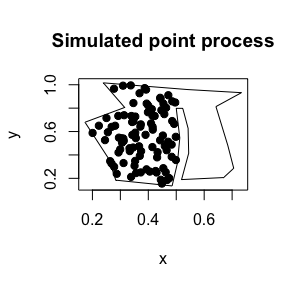out <- crossvalDensity(formLatticeOutput,PointPattern=Pointdata,
M=0.5,max_steps = 150)
plot(1:150,out$ucv,type="l")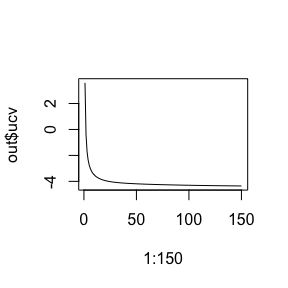out$k
##  150

The minimum uvc (Unbiased CrossValidation criterion of Sain, Baggerly and Scott (1994)) was at 150 steps. I’ll use that many steps to get a final, smooth density estimate using createDensity.

densityOut <- createDensity(formLatticeOutput,
PointPattern=Pointdata, k=out$k,intensity=FALSE, sparse = TRUE) #plot(densityOut) For the ecologically-inclined, the function homerange computes the area of the 95% confidence region. homerange(densityOut, percent=0.95)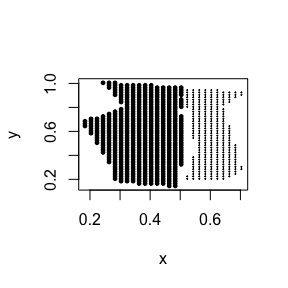## Proportion of region in homerange = 0.6605392 ## Area of homerange = 0.2142624 A Simple Kernel Example To illustrate the concept of kernels based on random walks, I’ll just compute the kernel for a simple network of nodes: Step One: x_poly = c(0, 0, 0.2, 0.2, 1, 1, 1.2, 1.2, 2, 2, 0) y_poly = c(0, 1, 1, 0.2, 0.2, 1, 1, 0.2, 0.2, 0, 0) polyg <- cbind(x_poly, y_poly) nodeFillingOutput <- nodeFilling(polyg, node_spacing=0.15) plot(nodeFillingOutput)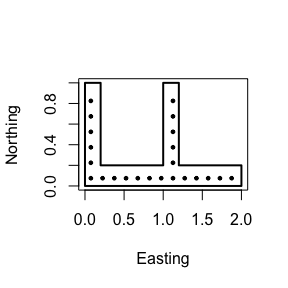I’ll sometimes refer to specific nodes, so for this illustration I’ll give them names: plot(nodeFillingOutput) text(nodeFillingOutput$nodes+rep(c(0.1,0),each=23),col=2,labels=letters[1:23])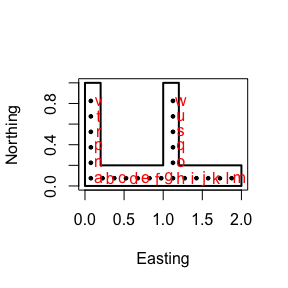Step Two: Create the lattice.

flo <- formLattice(nodeFillingOutput)
plot(flo)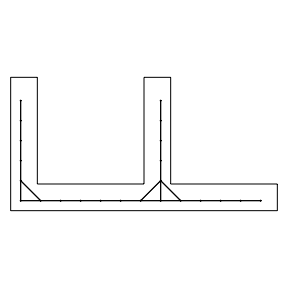Now we will add some observations at (1.5, 0.1), (1.5, 0.1) and (1.1, 0.5).

PD = rbind(c(1.5,0.1),c(1.6,0.1),c(1.1,0.5))
out <- createDensity(flo,PointPattern=PD,
M=0.5, k=1)
plot(out)
text(out$nodes, labels=round(out$probs,4),cex=0.6)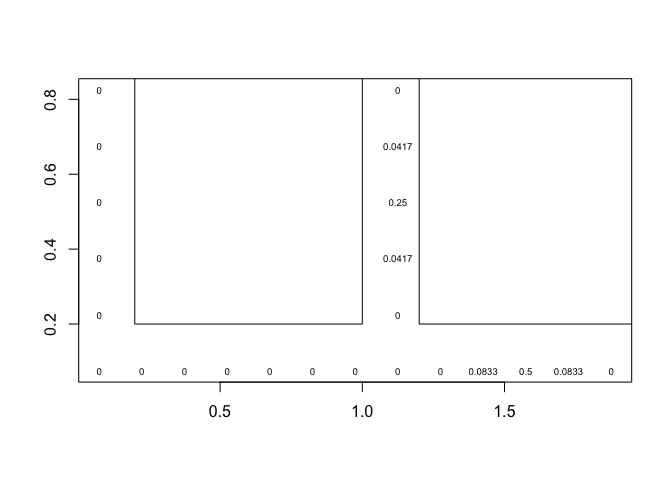I’ll go over this plot carefully. Note that we start with a density of 2/3 at node ‘k’ and 1/3 at node ‘s’, since those nodes are closest to the data locations. With M = 0.5 and a maximum (in this unusual case, since all the nodes are boundary nodes) of four neighbors (see node ‘o’), the flow rate is $$0.5/4 = 0.125$$ in all links. With $$k = 1$$, we see that there is now density $$0.125*(1/3) = 0.0417$$ at nodes ‘u’ and ‘q’, and density $$0.125*(2/3) = 0.0833$$ at nodes ‘j’ and ‘l’. Now have the distribution of a single-step random walk.

After many steps we will have a (nearly) uniform distribution of probabilities across the entire set of nodes:

out <- createDensity(flo,PointPattern=PD,
M=0.5, k=600)
plot(out)
text(out$nodes, labels=round(out$probs,3),cex=0.6)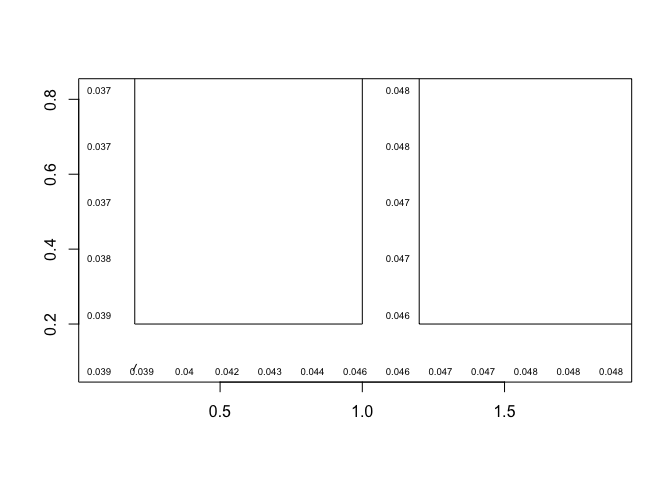Nonparametric Regression:

The density obtained from the latticeDensity smoother starting from a single observation is a bona fide probability distribution. These can be used as kernels for non-parametric surface estimation, using the Nadaraya-Watson estimator, which basically gets a prediction at each location by taking a weighted average of all observations, weighted by the value of the kernel started from the location of each observation. For a full disussion, see McIntyre and Barry (A Lattice-Based Smoother for Regions with Irregular Boundaries and Holes, Journal of Computational and Graphical Statistics, 2018, Vol. 27, pp. 360 - 367). All the user has to do is supply a polygonal boundary, observed values and their locations.

data(nparExample)
attach(nparExample)
plot.new()
#  Simulate a response variable
index1 = (grid2[,2]<0.8)|(grid2[,1]>0.6)
Z = rep(NA,length(grid2[,1]))
n1 = sum(index1)
n2 = sum(!index1)
Z[index1] <- 3*grid2[index1,1] + 4 + rnorm(n1,0,sd=0.4)
Z[!index1] <- -2*grid2[!index1,1] + 4 + rnorm(n2,0,sd=0.4)
#
coords=rbind(polygon2,polygon2[1,])
plot(coords,type="l")
points(grid2,pch=19,cex=0.5,xlim=c(-0.1,1))
text(grid2,labels=round(Z,1),pos=4,cex=0.5)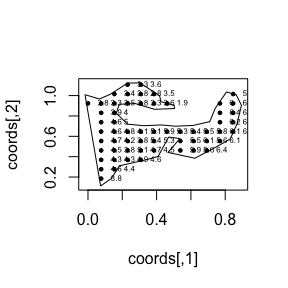nodeFillingOutput <- nodeFilling(poly=polygon2, node_spacing=0.025)
plot(nodeFillingOutput)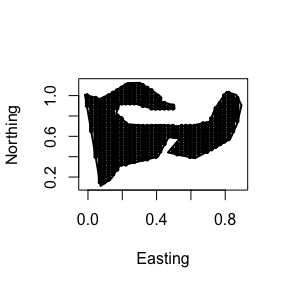formLatticeOutput <- formLattice(nodeFillingOutput)
plot(formLatticeOutput)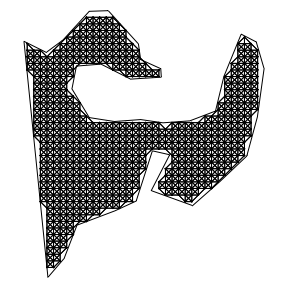NparRegOut <- createNparReg(formLatticeOutput,Z,PointPattern=grid2,k=4)
plot(NparRegOut)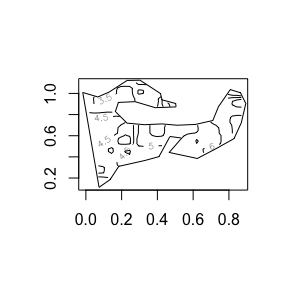names(NparRegOut)
##   "EW_locs"      "NS_locs"      "nodes"        "boundaryPoly"
##   "hole_list"    "PointPattern" "which_nodes"  "NparRegNum"
##   "NparRegDenom" "NparRegMap"   "sigma2"

Error map

The output of crossvalNparReg also includes an estiamate of the underlying error $$\hat{\sigma}^2 = \sum_{i=1}^n{(y_i-\hat{y}_{i(i)})^2/n}$$, which is the mean of the squared deleted residuals at all observations. In this case it equals 0.3248834.

You can also plot a countour map of spatial standard errors based on the Nadaraya-Watson kernel regression variance estimator. To do this, use the functions varianceMap and plot.varianceMapOut. Note that the standard error is not necessarily a good measure of mean squared prediction error, as the kernel estimator (especially when extrapolating in a part of the region far from the observations) can be biased.

varianceMapOut <- varianceMap(formLatticeOutput,Z,PointPattern=grid2,k=20)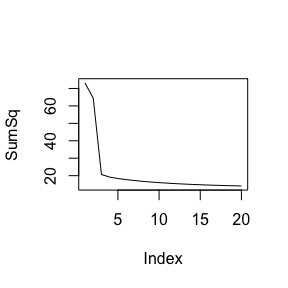plot(varianceMapOut)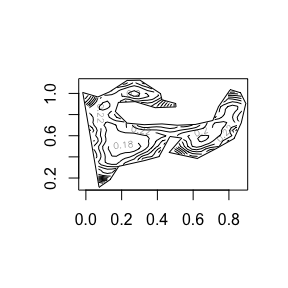Special cases:

Conversion to UTM

Shapefiles (and boundaries in general) are often in latitude and longitude. However, because the distance corresponding to one degree of latitude is usually not the same as the distance corresponding to one degree of longitude, the diffusion modeled by latticeDensity isn’t isotropic. To solve this problem, you can convert to UTM (Universal Transverse Mercator) coordinates. One R package that can do this conversion is rgdal:

library(rgdal)
xy <- cbind(c(118, 118.3, 118.7, 119), c(10, 25, 48, 49, 50))
## Warning in cbind(c(118, 118.3, 118.7, 119), c(10, 25, 48, 49, 50)): number
## of rows of result is not a multiple of vector length (arg 1)
now_in_UTM <- project(xy, proj = "+proj=utm +zone=51 ellps=WGS84")
now_in_UTM
##           [,1]    [,2]
## [1,] -48636.65 1109577
## [2,]  25379.91 2773187
## [3,] 179270.67 5325252
## [4,] 207462.87 5435168
## [5,] 141743.63 5550619

Holes

Islands inside of lakes and other ‘holes’ are easily accomodated in latticeDensity. Each polygonal hold is stored in a different object, then the corresponding nodes are cut out of the irregular region in the nodeFilling function.

boundary <- cbind(c(0,0,0.2,1,1),c(0,0.8,1,1,0))
hole1 <- cbind(c(0.1,0.3,0.4),c(0.2,0.3,0.8))
hole2 <- cbind(c(0.8,0.9,0.9),c(0.6,0.7,0.95))
region <- nodeFilling(poly = boundary, node_spacing = 0.03, hole_list = list(hole1, hole2))
##  "This function does not check to see if the holes"
##  "are nonintersecting, or whether they are contained"
##  "inside the boundary"
plot(region)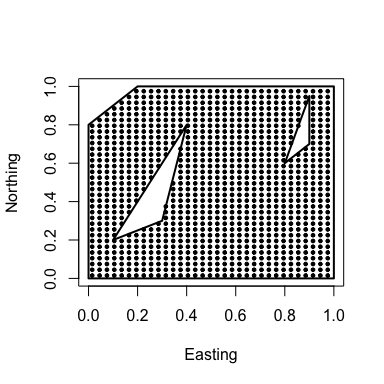lattice_output = formLattice(region)
plot(lattice_output)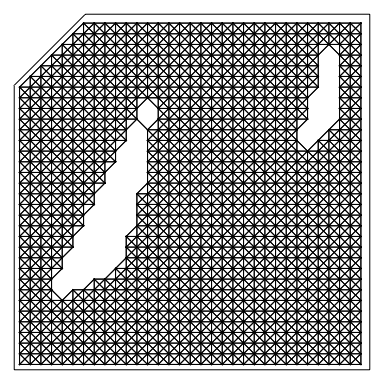There are some links that are crossing the islands, but the function editLattice can be used to remove these.

NOTE: Warnings

Occasionally the message

validspamobject() is deprecated. Use validate_spam() directly

will occur. This is generated by the spam package or packages that call spam and does not seem to have an effect on the output of latticeDensity.

Also, expect that some functions (crossvalidation or deletedResid, for instance) will not run properly if you only have one or two observations.

Finally, whenever a location is outside the support of all kernels, it is given the estimated value equal to the mean of the data. Usually it is better to make the kernels cover most of the map. Usually k is reasonably large compared to the diameter of the grid, and you are fine, otherwise you might consider decreasing the movement rate by decreasing M somewhat before crossvalidating. This will tend to make the kernels have larger support.

In a similar vein, crossvalidation often seems to undersmooth data when the observations are in clusters, a common problem with spatial crossvalidation in any context. If you think the optimum number of steps (k) under crossvalidation is too low, just use a larger value of k and examine the deleted sums of squared errors to see that they don’t increase too much.